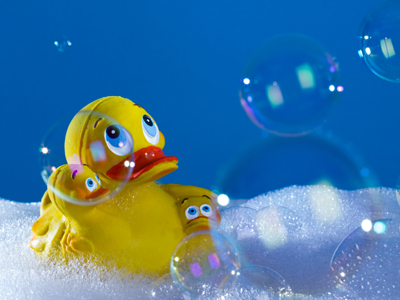How long will it take 80 litres of water in a bath to empty?

# Measures 3 (Difficult)

Welcome to the third in our difficult series of Eleven Plus maths quizzes on Measures. By now you will be very confident when it comes to converting metric units to Imperial ones, and vice versa. But how good are you at solving problems with different units of measurement? We’re about to find out!

Some of these questions may seem very hard at first glance but, if you break them down (and if you remember what you learned in the previous quizzes!), they aren’t that difficult really. Just use your common sense and you should be okay.

As always, take your time and read each question thoroughly before you choose your answers. And don’t forget to read the helpful comments after the questions. They explain how the problem was solved and give you tips and advice which you mighty later rely on in your exams. Good luck!

1.
Sarah’s dad is 6 feet tall. If 1 inch = 2.54 cm, how tall is Sarah’s dad in cm (to the nearest cm)?
153 cm
163 cm
173 cm
183 cm
To work this one out you need to know that there are 12 inches in a foot. So, 6 feet = 12 x 6 = 72 inches.
Now we just convert 72 inches to cm by multiplying by 2.54: 72 x 2.54 = 182.88 which we round up to 183 cm
2.
1 ounce = 28.34 g. What is the symbol for ounces?
on
oc
oz
ou
There are 16 oz (ounces) in 1 lb (pound)
3.
Stephen’s garden pond is 4 feet deep, 6 feet long and 5 feet wide. How much water will Stephen need to fill his pond?
Tip: 1 litre = 0.0353 cubic feet
120 litres
410 litres
1,440 litres
3,399 litres
First we must work out the volume of Stephen’s pond in cubic feet: 4 x 6 x 5 = 120 feet3
Next we convert 120 feet3 into litres by dividing by 0.0353: 120 ÷ 0.0353 = 3,399 litres
4.
If 1.61 km = 1 mile, how many miles is 783 kilometres (to the nearest mile)?
1,261 miles
486 miles
842 miles
386 miles
To convert km to miles just divide by 1.61: 783 ÷ 1.61 = 486.335 which we round down to 486 miles
5.
Marc weighs 45 kg but his mother only understands weight in stones and pounds. If 1 kg = 2.2 pounds, and there are 14 pounds in a stone, what is Marc’s weight in stones and pounds?
7 stones and 1 pound
6 stones and 11 pounds
8 stones and 7 pounds
7 stones and 5 pounds
First we convert 45 kg into pounds by multiplying by 2.2: 45 x 2.2 = 99 pounds.
Next we convert 99 pounds into stones by dividing by 14: 99 ÷ 14 = 7 remainder 1. Marc weighs 7 stones and 1 pound
6.
A tanker is loaded with 40,000 litres of petrol. It drops 15,000 litres off at one petrol station, and 12 million millilitres at a second. How much petrol is left inside the tanker?
13,000 litres
27,000 litres
34,800 litres
23,800 litres
12 million millilitres = 12,000 litres: just divide by 1,000. Now, add the volumes together, then subtract from 40,000: 15,000 + 12,000 = 27,000. 40,000 - 27,000 = 13,000 litres
7.
What is the name of the imperial unit of area, approximately equal to 4,047 m2?
A hectare
An acre
A chain
A furlong
An acre is 1640 of a square mile and equal to 0.405 hectares
8.
How many cubic inches are there in 1 cubic foot?
24 inches3
72 inches3
144 inches3
1,728 inches3
There are 12 inches in a foot, so there are 12 x 12 x 12 cubic inches in a cubic foot: 12 x 12 x 12 = 1,728
9.
The average person eats 900 kg of food a year. How many lbs of food is that if 1 pound = 0.454 kg?
3,964
2,973
1,982 lbs
991 lbs
To convert kg into lbs we must divide by 0.454: 900 ÷ 0.454 = 1,982 lbs
10.
A bath contains 80 litres of water. The plug is removed, and the water goes down at a rate of 250 ml per second. How long will it take for the bath to empty?
20 seconds
2 minutes and 20 seconds
5 minutes and 20 seconds
8 minutes and 20 seconds
First convert 80 litres into ml: 80 x 1,000 = 80,000
Next, divide 80,000 by 250: 80,000 ÷ 250 = 320 seconds.
Finally, convert 320 seconds into minutes: 320 ÷ 60 = 5 remainder 20. It will take 5 minutes and 20 seconds for the bath to empty
Author:  Frank Evans